# Operating Characteristics of Coupled-Cavity Semiconductor Lasers

This is a continuation from the previous tutorial - coupled-cavity semiconductor lasers.

In this tutorial we present the experimental results for coupled-cavity lasers with particular attention paid to active-active $$\text{C}^3$$ devices.

The properties considered are longitudinal-mode selectivity under CW operation, wavelength tuning with current adjustment, domains of single-mode operation under modulation, and the frequency chirp.

A few transmission experiments employing $$\text{C}^3$$ lasers are also briefly described. Finally, we discuss the performance of external-cavity semiconductor lasers and their potential applications in optical fiber communications.

## 1. Longitudinal-Mode Control

Figure 8-8 shows an example of the $$L-I$$ curves obtained at room temperature under CW operation of a 1.5-μm CSBH $$\text{C}^3$$ laser. The inset shows the biasing scheme. $$I_\text{L}$$ and $$I_\text{R}$$ are the bias currents for the left and right sections.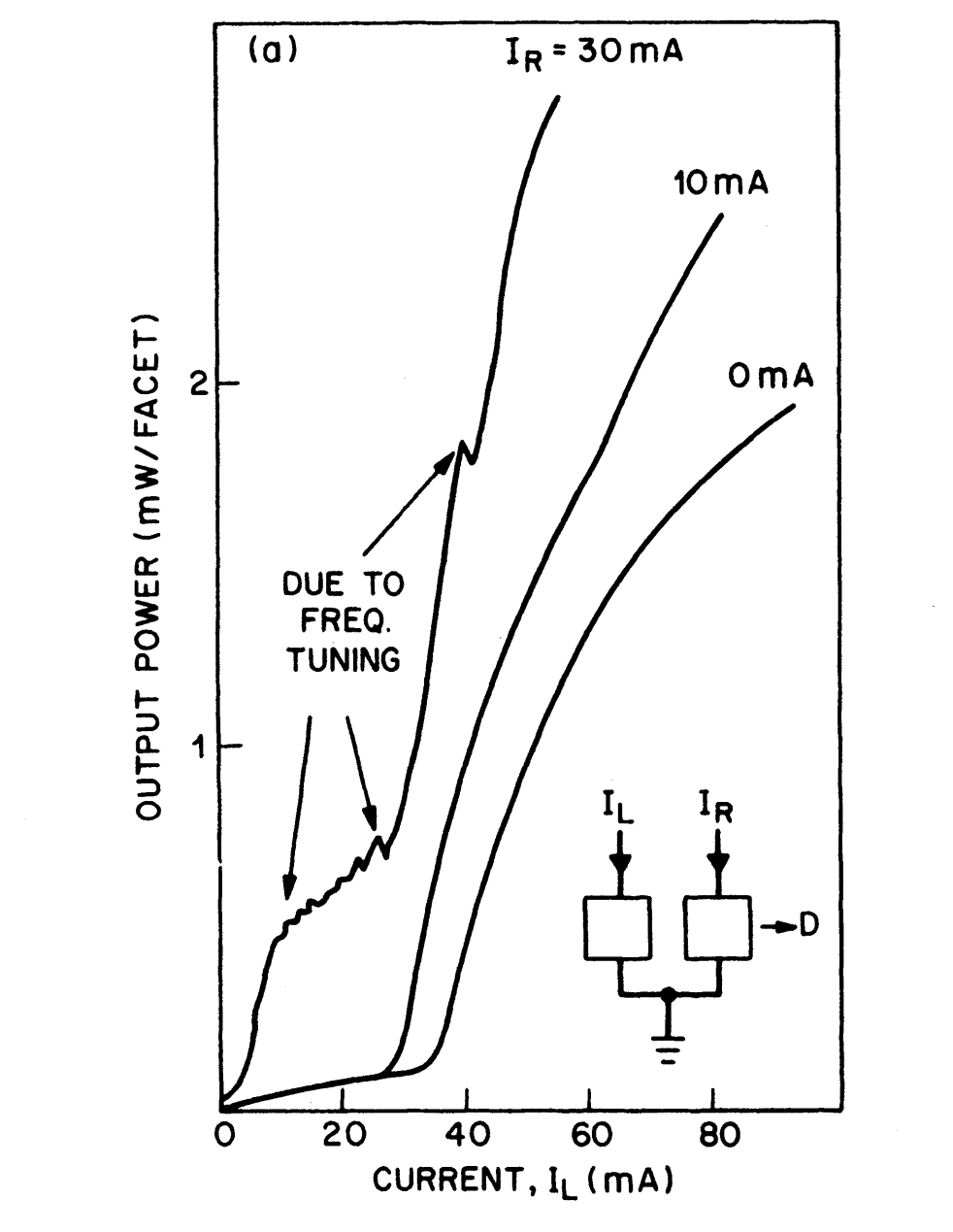Figure 8-8.  Light-current characteristics of a $$\text{C}^3$$ laser for different biasing of the right section. Inset shows the biasing scheme and the position of the detector (D). As the right-cavity current $$I_\text{R}$$ increases, the threshold decreases because of reduced absorption in the right cavity. When $$I_\text{R}$$ exceeds the threshold value (20 mA), the right cavity acts as a laser while the left-cavity acts as controller. The bumps in the $$I_\text{R}=30\text{ mA}$$ curve represent hopping of the laser wavelength to successive longitudinal modes.

When $$I_\text{R}\lt20\text{ mA}$$ (the threshold current of the right section), the $$L-I$$ curves for the left section are similar to those obtained for single-cavity lasers. An increase in $$I_\text{R}$$ decreases the threshold since the right diode becomes less lossy.

However, when $$I_\text{R}\gt20\text{ mA}$$, the $$L-I$$ curves show qualitatively different behavior, with a ripple-like structure below threshold. Some ripples or kinks also occur in the above-threshold regime but are more widely separated when compared to those occurring below threshold. Spectral measurements show that these kinks in the $$L-I$$ curve are associated with the currents at which the laser switches from one longitudinal mode to another one.

The observed behavior can be understood physically as follows.

When $$I_\text{R}=30\text{ mA}$$ in Figure 8-8, the right cavity is acting as the laser while the left cavity takes the role of controller.

When biased below threshold, the controller acts as a frequency selector. A change in its current changes the carrier density. This in turn changes the refractive index, which results in a shift of the FP modes of the controller with respect to the laser cavity FP modes.

A shift of the controller FP modes manifests as a shift of the loss profile in Figure 8-1 [refer to the coupled-cavity semiconductor lasers tutorial]. When the shift is large enough so that the lowest loss occurs for the next laser-cavity FP mode, mode hopping occurs.

This longitudinal-mode hopping is manifested by the observed ripples in the $$L-I$$ curve. The discrete mode hops are sometimes referred to as wavelength tuning.

During each hop the wavelength shifts by one FP-mode spacing (~ 2 nm for a 150-μm-long cavity), and a tuning range of more than 10 nm is readily achieved. The exact number of mode hops is determined by the relative cavity lengths, as discussed in the Longitudinal Modes and Threshold Gain section of the coupled-cavity semiconductor lasers tutorial.

Figure 8-9 shows the longitudinal mode spectra of a CSBH $$\text{C}^3$$ laser that showed a tuning range of 26 nm through 13 discrete mode hops of about 2 nm each. This is one of the largest tuning ranges achieved with the use of the coupled-cavity scheme.

Also shown in the figure is the current change $$\Delta{I}_\text{L}$$ needed for successive mode hops plotted as a function of laser wavelength. Small values of $$\Delta{I}_\text{L}$$ are necessary when the controller is biased considerably below threshold.

However, as $$I_\text{L}$$ increases, the value of $$\Delta{I}_\text{L}$$ needed for the next mode hop increases rapidly. This is so because the carrier density begins to saturate as the controller approaches its threshold.

Nonetheless, for the $$\text{C}^3$$ laser shown in Figure 8-9, the wavelength shifted by about 26 nm when the modulator current was changed by 11.6 mA. This resulted in an average tuning rate of more than 2 nm/mA.

The current step $$\Delta{I}_\text{L}$$ required for a mode hop also depends on the relative cavity lengths $$L_1$$ and $$L_2$$. The long-long geometry ($$L_1\approx{L}_2$$) was used in obtaining Figures 8-8 and 8-9.

For a long-short device, much larger values of $$\Delta{I}_\text{L}$$ than shown in Figure 8-9 would be required since the FP mode of the short cavity has to shift by one mode spacing of the long cavity for a mode hop to occur.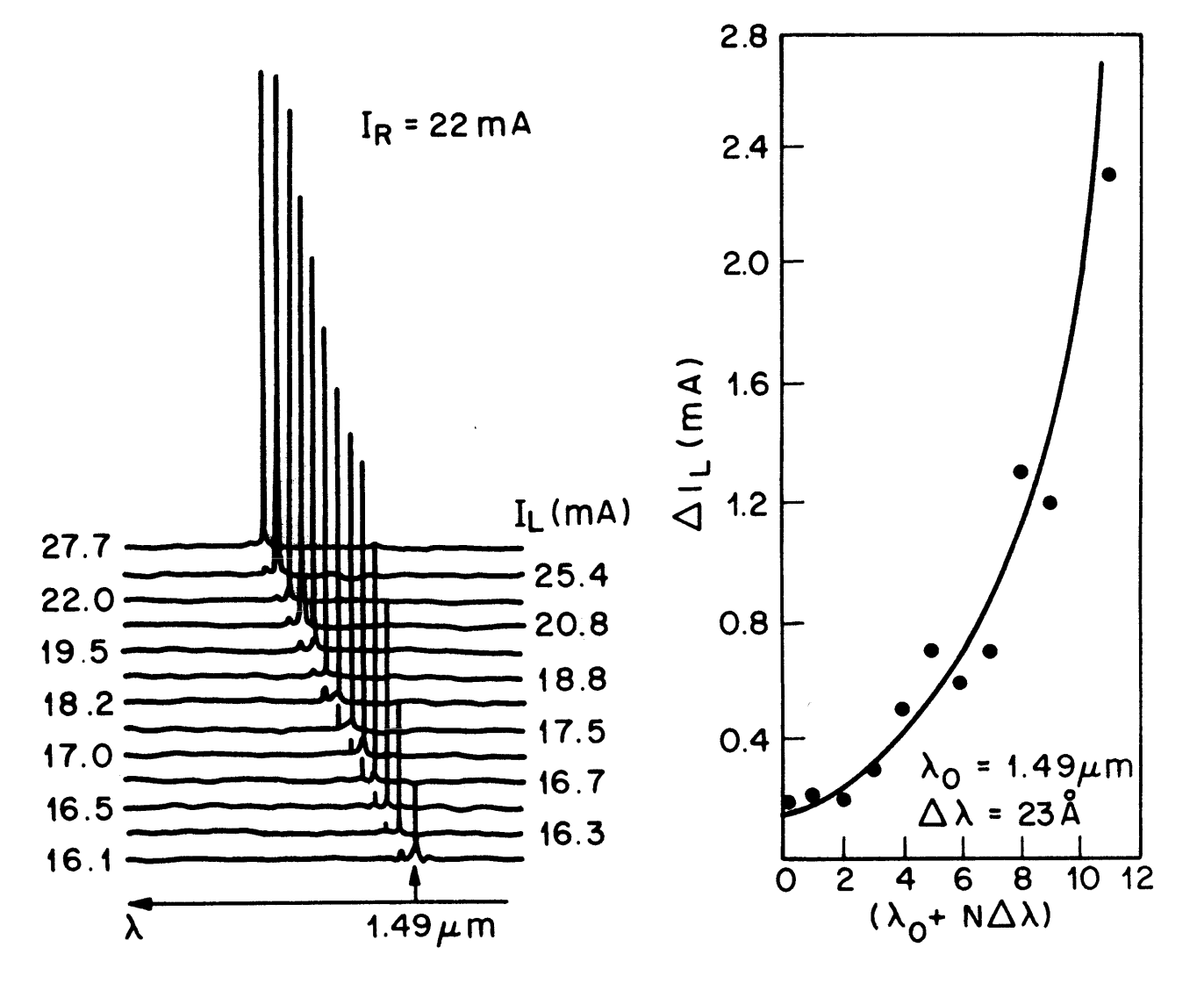Figure 8-9.  Wavelength tuning of a $$\text{C}^3$$ laser using the biasing scheme shown in the inset of Figure 8-8. Successive longitudinal modes of the right cavity (acting as the laser) can be selected by changing the current $$I_\text{L}$$ in the left controller section. The current change $$\Delta{I}_\text{L}$$ as a function of the mode number $$N$$ is also shown with solid dots. The solid curve shows the trend of $$\Delta{I}_\text{L}$$ increasing with $$N$$.

When the controller is operating above threshold, the carrier density is approximately pinned and its FP modes stop shifting with an increase in $$I_\text{L}$$. Under ideal conditions no mode hopping is expected.

In practice, however, occasional mode hoping occurs because of a partial clamping of the carrier density or because of temperature-induced index variations.

Nonetheless, as Figure 8-8 shows, when both sections of a $$\text{C}^3$$ laser are biased above threshold, the device is able to maintain the same longitudinal mode over a considerable range of current. This mode of operation is useful from the standpoint of wavelength stability.

## 2. Optimum Biasing for Direct Modulation

From the viewpoint of applications to optical fiber communications, it is essential that the laser maintains the same longitudinal mode under high-speed direct modulation while at the same time the side modes remain reasonably suppressed (by more than 20 dB) throughout the modulation cycle.

The $$L-I$$ curves shown in Figure 8-8 indicate that a $$\text{C}^3$$ laser behaves differently depending on whether the controller section is biased below or above threshold. As the currents in the two cavity sections are varied, the $$\text{C}^3$$ laser has domains of single-longitudinal-mode operation.

Figure 8-10 shows these domains relative to the currents applied to each section for a 1.55-μm ridge waveguide $$\text{C}^3$$ laser.

The dotted line corresponds to the threshold. Regions $$\text{A}$$ and $$\text{B}$$ are the tuning regions corresponding to the situation where one section is biased above its lasing threshold while the other section (controller) is biased below threshold all the time.

The horizontal lines in region $$\text{A}$$ and the vertical lines in region $$\text{B}$$ are the boundaries along which the laser switches abruptly from one longitudinal mode to the adjacent one. Within each narrow stripe the laser maintains the same longitudinal mode.

In the square-shaped region $$\text{C}$$, both cavities are biased above threshold and the same longitudinal mode is maintained throughout this region.

Both sections are below threshold in the region $$\text{D}$$.

Dashed lines in Figure 8-10 show the biasing schemes employed for various modes of operation of a $$\text{C}^3$$ laser.

The line 1 corresponds to wavelength tuning. The laser cavity is biased above threshold ($$I_1\gt{I}_1^\text{th}$$) and $$I_2$$ is varied such that the controller is below threshold. The wavelength-tuning regime has already been discussed and illustrated by Figures 8-8 and 8-9.

Line 2 corresponds to analog direct-frequency modulation. Here one section, say cavity 2, is biased above threshold while cavity 1 acts as the controller ($$I_1\lt{I}_1^\text{th}$$). A small modulation current is applied to the controller such that the laser operates within the narrow stripe region without mode hops. Carrier-density variations in the controller section leads to the periodic modulation of the laser frequency around its bias value.

Modulation amplitudes of ~ 10 GHz/mA are easily accomplished. This should be contrasted with the single-cavity case where frequency modulation is typically less than 1 GHz/mA and, furthermore, is always accompanied by spurious intensity modulation. For a $$\text{C}^3$$ laser the intensity remains almost constant since the laser bias remains unchanged.

For the intensity modulation commonly employed in optical communication systems, there are two biasing schemes.

One can operate in regions $$\text{A}$$ or $$\text{B}$$ of Figure 8-10 after applying the optimum bias current to the controller (say cavity 1) so that the $$\text{C}^3$$ laser is operating within one of the narrow stripes at a point where the MSR is highest. The current in cavity 2 is then modulated as shown by the operation line 3.

In the other mode of operation, both cavities are biased above threshold so that the laser is operating within the square region $$\text{C}$$, where it maintains the same longitudinal mode throughout modulation. In order to avoid entering the mode-hopping regions $$\text{A}$$ and $$\text{B}$$, the modulation current may be split between the two cavities such that the resulting operation line is tilted in the $$I_1-I_2$$ plane. This is illustrated in Figure 8-10 through dashed lines 4 and 5, whose lengths denote the peak-to-peak amplitudes of the modulation current while the solid dot in the center of each line corresponds to the bias level.

The choice between these two biasing schemes for direct intensity modulation depends on several factors.

To start with, the side modes should remain reasonably suppressed throughout a modulation cycle. For the $$\text{C}^3$$ laser of Figure 8-10, the MSR was measured at different operating points giving the values shown. Although it was slightly higher in region $$\text{C}$$, MSR values of more than 20 dB were measured in all three regions. Some $$\text{C}^3$$ lasers have shown MSR values exceeding 30 dB.

The other criterion is related to the mode stability; i.e., the laser should remain at the same longitudinal mode under external perturbations such as temperature fluctuations. In this respect the biasing of a $$\text{C}^3$$ laser in the region $$\text{C}$$ is preferable simply because the single-mode domain is wider.

For some applications such as wavelength multiplexing, it is desirable to have a semiconductor laser whose wavelength is selected by tuning and then remains stable under modulation.

This would require the operation of a $$\text{C}^3$$ laser in the tuning regime. The wavelength stabilization can then be achieved through a feedback loop that automatically adjusts the bias currents to maximize the MSR.

A powerful technique for stabilization and optimum biasing of $$\text{C}^3$$ lasers consists of plotting the constant power curves in the $$I_1-I_2$$ plane. These curves can be used to determine the bias and modulation currents for the two cavities. A feedback loop can provide stable operation over wide ranges of temperature and power.

## 3. Frequency Chirp

The mode-discrimination ability of coupled-cavity lasers inherent in their design results in single-frequency lasers that oscillate predominantly in a single longitudinal mode with side modes suppressed by 20-35 dB. Furthermore, in contrast to single-cavity lasers, these side modes remain suppressed even when the coupled-cavity laser is directly modulated at frequencies in the gigahertz range.

The dynamic spectral width of such a laser is then determined by frequency chirping. Similar to the case of DFB lasers, the frequency chirp is expected to be the limiting factor in the performance of optical communication systems employing coupled-cavity lasers.

Considerable attention has been paid to the measurement and minimization of frequency chirp in coupled-cavity lasers. The measurements for $$\text{C}^3$$ lasers operating at 1.3-μm and 1.55-μm wavelengths have shown that the coupled-cavity nature of these lasers not only provides mode selectivity but is also helpful in reducing the chirp.

Figure 8-11 shows experimental results where dashed lines show the corresponding behavior for a single-cavity laser.

To ensure that the chirp reduction is mainly due to the coupled-cavity mechanism, two lasers were selected from the same wafer and one of them was converted to a $$\text{C}^3$$ laser by cleaving it approximately in the middle. Both sections were biased above threshold to give about 2 mW of power and were modulated sinusoidally at 100 MHz.

The chirp varies almost linearly with the modulation-current (peak-to-peak) amplitude for both single-cavity and coupled-cavity lasers. However, at a given modulation current the chirp for a coupled-cavity laser is typically reduced by a factor of 2 compared with its value for the single-cavity laser.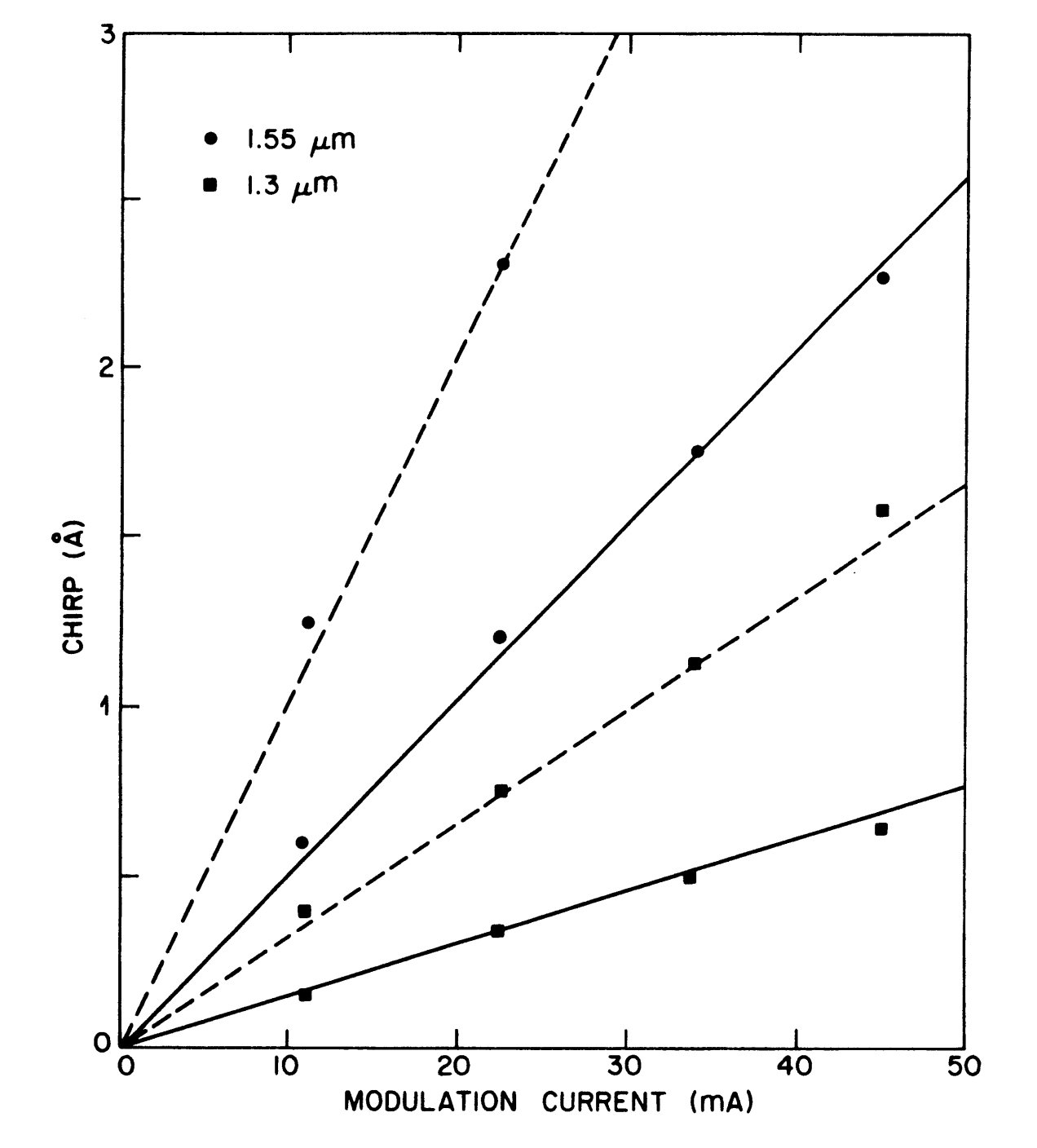Figure 8-11. Variation of the chirp with the modulation current (solid lines) for two $$\text{C}^3$$ lasers oscillating at 1.3- μm and 1.55-μm wavelengths. For comparison, dashed lines show the chirp behavior of a conventional single-cavity semiconductor laser obtained from the same wafer.

The exact amount of chirp reduction for a specific coupled-cavity laser depends on a large number of parameters such as the modulation frequency, the bias levels, and the fractions into which the modulation current is split for the two cavity sections.

Some of the parameters can be optimized to obtain the minimum chirp.

For a long-long $$\text{C}^3$$ device, both cavity sections are often biased above the threshold for the purpose of direct modulation. In this case the bias levels $$I_1\text{b}$$, $$I_2^\text{b}$$, and the modulation-slitting fraction can be optimized for chirp minimization.

The small-signal analysis for this case reveals that the chirp is minimum when both sections have equal relative bias levels ($$I_1^\text{b}/I_1^\text{th}\approx{I}_2^\text{b}/I_2^\text{th}$$) and when the modulation current is split equally between them.

The numerical results [see Figure 8-7 in the coupled-cavity semiconductor lasers tutorial] also show that chirp reduction depends on the strength of intercavity coupling, on the coupling phase, and on the modulation frequency.

For a long-short device, chirp measurements have been carried out when the short section (section 2) is biased below threshold.

For a given bias $$I_2^\text{b}$$ less than $$I_2^\text{th}$$, the modulation-splitting fraction $$m_\text{s}$$ and the relative phase $$\theta_\text{m}$$ [see Equation (8-3-24) in the coupled-cavity semiconductor lasers tutorial] were adjusted to minimize the chirp.

For small values of $$I_2^\text{b}$$, small values of $$m_\text{s}$$ of about 0.1 were required. However, when $$I_2^\text{b}$$ was increased considerably, the optimum chirp occurred when the modulation current was split equally without phase shift between the two sections.

## 4. Transmission Experiments

Similar to the case of DFB lasers, the ability of coupled-cavity semiconductor lasers to operate stably in a single longitudinal mode can be exploited for use in optical communication systems at 1.55 μm where conventional silica fibers have lowest losses but suffer from a relatively large chromatic dispersion of $$15-18\text{ ps}/(\text{nm}\cdot\text{km})$$. Both the active-passive (external cavity) and active-active schemes have been used for this purpose.

In this section, we briefly describe the results of transmission experiments employing $$\text{C}^3$$ lasers.

In one transmission experiment a ridge waveguide $$\text{C}^3$$ laser at 1.55 μm was used to transmit signals over 161.5 km of single-mode fiber at the bit rate of 420 Mb/s. The temperature was held at 20.1°C. The controller section of the $$\text{C}^3$$ laser was biased below threshold at 12.1 mA. The laser-cavity current was switched between 43.9 and 94.9 mA to generate a pseudorandom bit stream in the NRZ format. The average power launched into the fiber was 0.9 dBm.

The fiber output was coupled to an InGaAs avalanche photodetector with an optimized average gain of 9.1. The receiver sensitivity for a bit rate error rate of $$10^{-9}$$ at 420 Mb/s was $$-42.6$$ dBm. A error rate of $$5\times10^{-10}$$ was obtained after passing the signal through a 162-km-long fiber with an average received power of $$-41.7$$ dBm. Figure 8-12 shows the measured bit error rate as a function of the received power for fibers 10 km and 162 km long. A direct comparison shows a dispersion penalty of only about 0.7 dB even though the fiber had a dispersion of $$17\text{ ps}/(\text{nm}\cdot\text{km})$$ at the 1.55-μm wavelength used in the experiment. This penalty may be attributed to the wavelength chirp.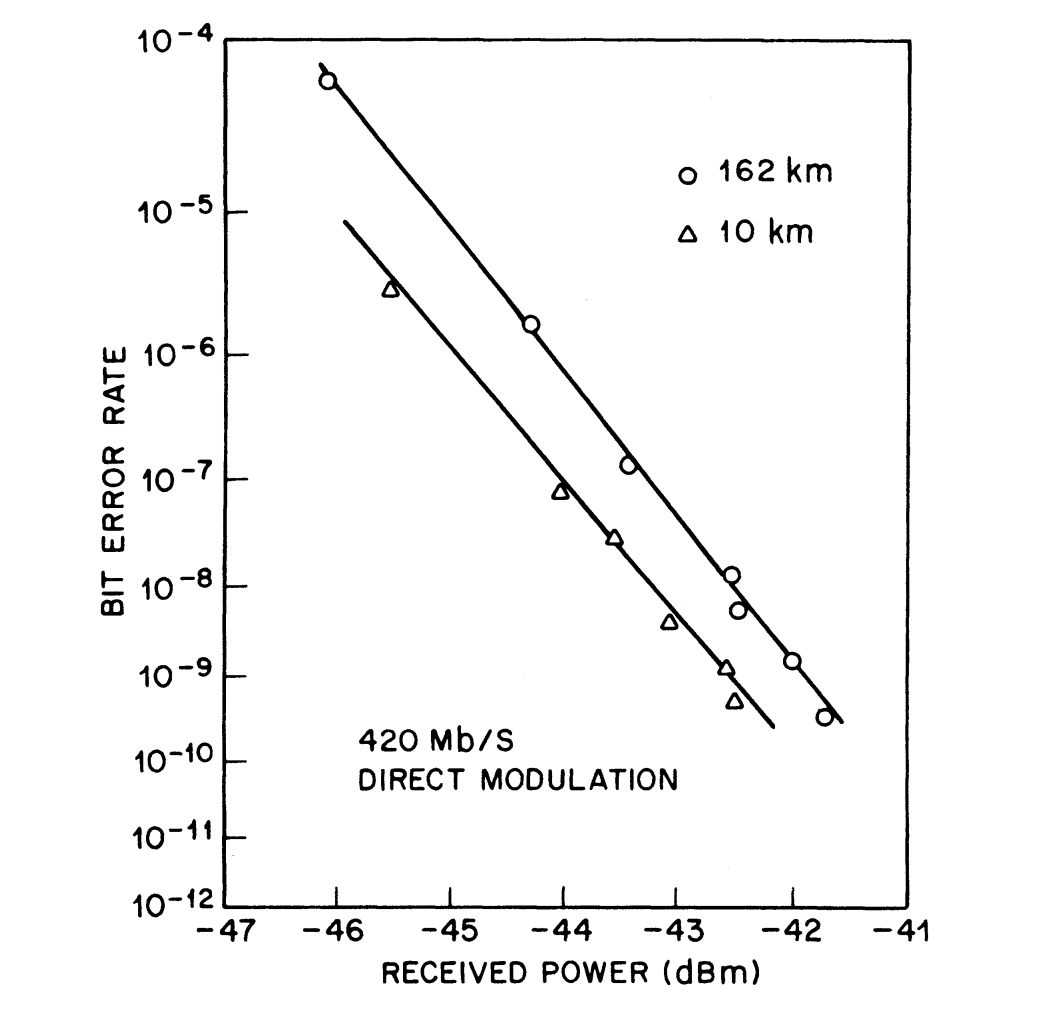Figure 8-12.  Bit error rate as a function of received power. Results of a transmission experiment employing a $$\text{C}^3$$ laser modulated at the bit rate of 420 Mb/s over fibers 10 and 162 km long.

Another transmission experiment used a similar 1.55-μm ridge waveguide $$\text{C}^3$$ laser to increase the bit rate to 1 Gb/s. A bit error rate of $$1\times10^{-10}$$ was obtained after transmission through a 101-km-long fiber with an average received power of $$-16.2$$ dBm. The receiver sensitivity was limited because of the use of a PIN-diode photodetector. The use of an avalanche photodetector led to an increase in the fiber length to 120 km at the bit rate of 1 Gb/s. To minimize chirp, it was necessary to operate the laser with an on-off ratio of 3.7:1. Such a low extinction ratio resulted in a power penalty of about 4.5 dB.

In these transmission experiments the wavelength chirp was a limiting factor. To increase the bit rate even further, it is necessary to reduce the chirp. One approach eliminated the chirp problem altogether by using an external modulator. Since the semiconductor laser uses CW operation, the modulation-induced line broadening does not occur, and the source bandwidth is just the CW line width of the single longitudinal mode maintained by the device. The potential of this approach was demonstrated in 1985 by a 117-km transmission experiment at the bit rate of 4 Gb/s. A 1.53-μm CSBH $$\text{C}^3$$ laser was used in the experiment, and the laser exhibited a mode suppression ratio of 5000. A Ti:LiNbO3 traveling-wave directional coupler switch served as the external modulator with an on-off ratio of 12:1. Figure 8-13 shows the measured bit-error-rate curves with and without fiber. A slight power penalty of about 0.5 dB occurring for the 117-km-long fiber is attributed to the finite dispersion [~ $$18\text{ ps}/(\text{nm}\cdot\text{km})$$] of the fiber used in the experiment and represents the intrinsic information-bandwidth limitation.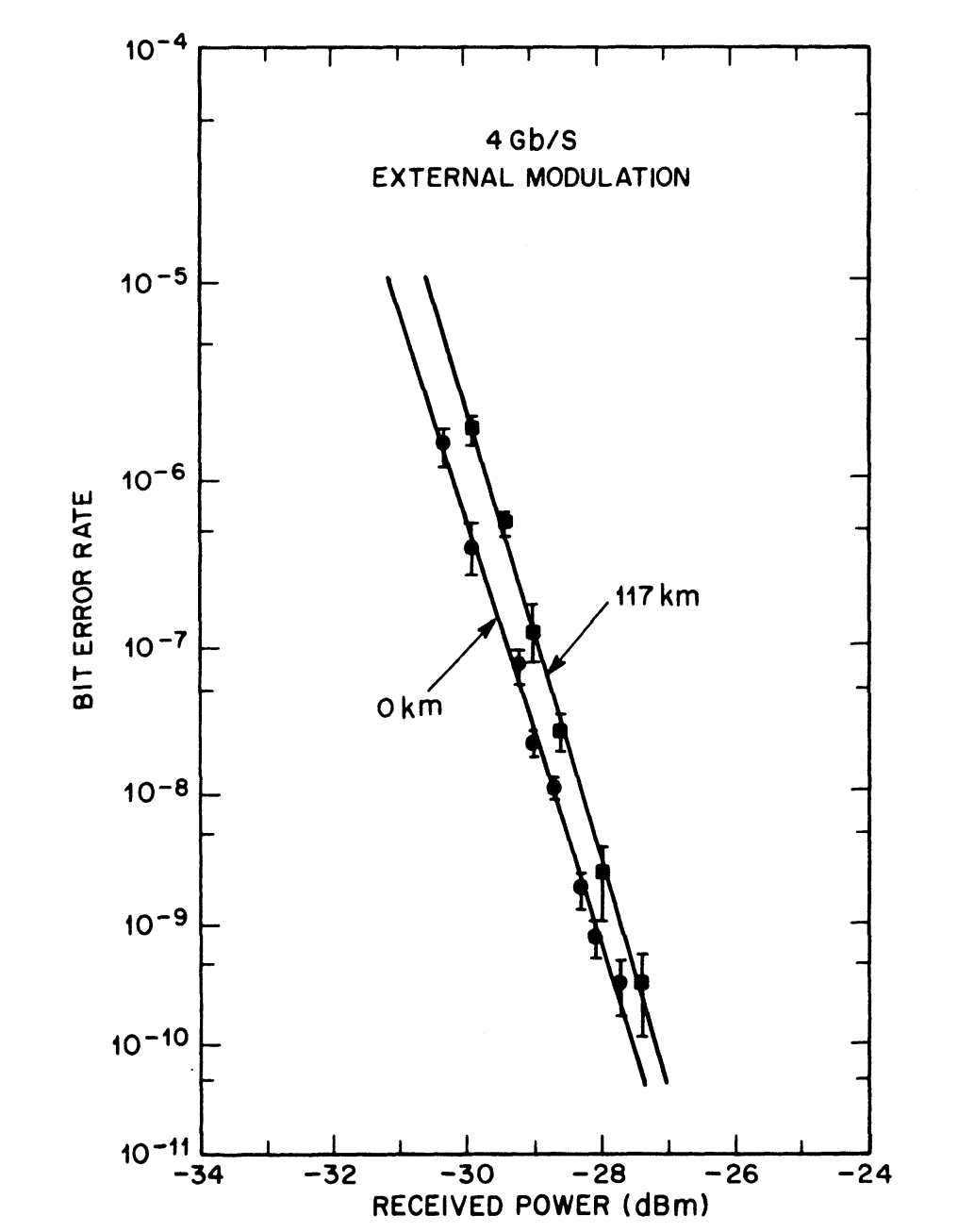Figure 8-13.  Bit-error-rate curves for a 117-km transmission experiment employing a CW-operated $$\text{C}^3$$ laser with 4 Gb/s of external modulation.

In spite of their record performance in the 1985 transmission experiment, interest in $$\text{C}^3$$ lasers declined because they had problems related to mode hopping and stability under field conditions. Tunable DFB and DBR lasers were developed to meet the somewhat conflicting requirements of wavelength tuning and stability. Some coupled-cavity semiconductor lasers have continued to be attractive; they are external-cavity lasers belonging to the active-passive class, and the next section discusses their performance.

## 5. External-Cavity Semiconductor Lasers

So far we have considered the operating characteristics of active-active devices. As mentioned before, the active-passive scheme, where an external cavity is used to control the longitudinal modes of a conventional semiconductor laser, also has potential applications in optical fiber communications and has attracted considerable attention.

In this section we briefly describe the performance of such external-cavity semiconductor lasers. Although the capability of longitudinal-mode control through an external cavity has long been known, it is only recently that the technique has been applied for InGaAsP lasers oscillating in the 1.3-1.6 μm wavelength range.

In one approach the external cavity was formed by placing a concave spherical reflector at a distance of about 200 μm from one laser facet. The use of confocal geometry resulted in significant coupling even when the laser facet was not antireflection-coated.

As discussed in the coupled-cavity semiconductor lasers tutorial, the coupled-cavity device selects the single longitudinal mode closest to the gain peak with the lowest cavity loss. Furthermore, the wavelength tuning can be achieved by changing the external-cavity length, which can be controlled either thermally or electronically (using a piezoelectric transducer in the latter case).

For a fixed external-cavity length, tuning can also be achieved by changing the temperature of the laser cavity. A temperature change alters the refractive index resulting in a change in the optical length; this in turn shifts the laser-cavity modes.

The tuning range or the number $$M$$ of the laser-cavity modes, over which the coupled-cavity laser can be made to hop discretely before returning to its original wavelength, depends on the relative cavity lengths.

Similar to the case of $$\text{C}^3$$ lasers, $$M\approx\mu_1L_1/\mu_2L_2$$ in the long-short geometry, where the external-cavity length $$L_2\ll{L}_1$$ and $$\mu_2\approx1$$ for the air-filled cavity. For a 250-μm-long laser, $$M=6$$ if $$L_2=150$$ μm, and a tuning range of 6 nm can be easily achieved.

The MSR also depends on the external-cavity length partly because, for a given radius of curvature of the spherical reflector, the intercavity coupling changes with $$L_2$$. However, MSR values of more than 30 dB can be achieved with proper design. The practical utility of spherical-mirror external-cavity design was demonstrated in an early 102-km transmission experiment at a bit rate of 140 Mb/s.

In a slightly different approach, a graded-index lens (GRINROD) with an antireflection coating at the front end and a reflective coating at the far end was used for the external cavity. The lens was epoxied inside a glass capillary tube, which in turn was held by a copper stud epoxied to a laser heat sink, as shown in Figure 8-2 in the coupled-cavity semiconductor lasers tutorial.

The GRINROD cavity was about 160 μm long, leading to the optical length ratio of 4:1 for the laser and external cavities. An advantage of the use of a GRINROD is that its focusing properties ensure significant optical coupling between the two cavities.

The performance of GRINROD external-cavity lasers was demonstrated in a 99-km transmission experiment at the bit rate of 1 Gb/s. A 1.55-μm ridge waveguide laser was coupled to the GRINROD as shown in Figure 8-2 [refer to the coupled-cavity semiconductor lasers tutorial].

The coupled-cavity laser exhibited an MSR of better than 30 dB. The average power launched into the fiber was 0.96 dBm and the on-off extinction ratio was 3.5:1. Despite a power penalty of approximately 3 dB caused by the low extinction ratio, a bit error rate of $$1\times10^{-9}$$ was obtained with a received power of $$-26.5$$ dBm.

Short coupled-cavity semiconductor lasers have also been shown to maintain the single-longitudinal-mode operation at modulation frequencies lying in the gigahertz range.

In this approach a short laser ($$L_1=50-80$$ μm) is coupled to a short ($$L_2=30-80$$ μm) air-filled cavity by simply placing a cleaved semiconductor chip with a gold-coated facet next to the laser on the common copper heat sink. The main advantage of short cavities is that the longitudinal modes are relatively far apart leading to an improved gain roll-off discrimination for the adjacent pairs of coincident modes [see Figure 8-1 in the coupled-cavity semiconductor lasers tutorial].

In these external-cavity schemes, mode selectivity arises from interference between the waves propagating in the two cavities. An additional mode-selective mechanism can be introduced if the feedback from the far end of the external cavity is dispersive, i.e., the reflectivity of the external mirror is wavelength-dependent. This can be achieved by using a grating or a properly designed frequency-selective filter. The use of a grating has the additional advantage that the laser wavelength can be tuned over a considerable range (~ 50 nm) by rotating the grating.

An aspect of coupled-cavity semiconductor lasers that has attracted considerable attention is related to their ability to exhibit a smaller CW line width compared with that of a conventional semiconductor laser.

The line width of a single-cavity semiconductor laser has been discussed in the noise characteristics of semiconductor lasers tutorial and is typically 100 MHz at a power level of 1 mW. For a $$\text{C}^3$$ laser or a GRINROD external-cavity laser with similar cavity lengths, this number can be reduced by about one order of magnitude.

In the case of external-cavity semiconductor lasers, however, the line width can be further reduced by increasing the length of the external cavity. The physical basis for the line-width reduction can be understood by using Equation (6-5-52) [refer to the noise characteristics of semiconductor lasers tutorial]. An increase in the total cavity length has the effect of increasing the photon lifetime. This results in a much larger number of intracavity photons at a given output power and, as shown by Equation (6-5-52) the line width of the single longitudinal mode decreases. Line widths as small as 10 kHz have been demonstrated using few-centimeter-long external cavities.

The performance of external-cavity semiconductor lasers can be considerably improved if a wavelength-selective element such as a grating is used in the external cavity.

The simplest scheme discussed earlier makes use of a mechanically ruled grating. However, distributed feedback can also be used to provide a Bragg grating that can be placed at the end of the external cavity.

In one scheme, the Bragg grating used to provide the distributed feedback is fabricated on a silicon chip. A rate-equation approach has been used to model such lasers in which the active section is coupled to a high-Q external cavity. It shows that the static frequency chirp is reduced by a factor that depends on the operating conditions. Under the same operating conditions the line width is reduced by the square of that factor. This relationship between the static chirp and the line width under CW operating has been verified experimentally.

Line widths below 0.5 MHz have been obtained, and even smaller line widths are possible. The dynamic performance of such external-cavity semiconductor lasers is also considerably improved. They are also capable of producing picosecond optical pulses through mode locking. In another approach the grating is etched directly onto a fiber that is coupled to the multimode laser. Such a fiber-grating laser has been used to produce 18.5-ps pulses at a repetition rate of 2.37 GHz.

External-cavity semiconductor lasers do not usually have a monolithic design, and this is a major limitation. It is possible to design a monolithic external-cavity semiconductor laser by forming the passive waveguide on the same substrate used for fabrication of the active section.

In one 1.55-μm device, the 0.6-mm-long Bragg mirror (acting as an integrated external grating) was separated from the 0.7-mm active region by a 4.2-mm passive waveguide, resulting in a 5.5-mm-long external-cavity semiconductor laser. All three components were formed on the same InP substrate to preserve the monolithic nature of the device. This laser produced 20-ps optical pulses at 8.1 GHz repetition rate through active mode locking.

In another implementation, a 1.2-mm-long laser provided 200-kHz line width at 17-mW operating power. The laser has a low current (8 mA) and could be operated up to 28 mW.

External-cavity semiconductor lasers have found applications in soliton communication systems. Soliton transmission over 15,000 km at 5 Gb/s and over 11,000 km at 10 Gb/s has been demonstrated by using the recirculating fiber-loop configuration and an external-cavity mode-locked laser. The highest bit rate of 32 Gb/s was demonstrated in 1992 in another soliton experiment that used a mode-locked external-cavity semiconductor laser.

The next tutorial discusses the diverse applications of coupled-cavity semiconductor lasers.